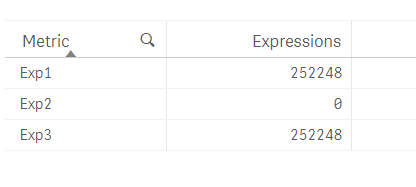# New to Qlik Sense

Discussion board where members can get started with Qlik Sense.

Announcements
Uploads getting stuck in the virus scanner. We are investigating.
cancel
Showing results for
Did you mean:
HighlightedCreator

## Aggr Expressions NOT Working when using ValueList() to create Synthetic Dimension

I have attached a sample Qlik Sense App that contains a straight table with Custom Dimensions and Expressions.

Here you would see that the second expression DOES NOT generate any output, even though it is the same expression. The only thing that stands out to me is that, the second instance of AGGR in such scenario doesn't work.

Front End TableTable Expression Code

Dimension:

=ValueList('Exp1', 'Exp2', 'Exp3')

Expression:

Pick(Match(ValueList('Exp1', 'Exp2', 'Exp3'), 'Exp1', 'Exp2', 'Exp3')

, Sum(Aggr(Sum(Expression1), Dim1))

, Sum(Aggr(Sum(Expression1), Dim1))

, Sum(Expression1)

)

Any help is appreciated.

1 Solution

Accepted Solutions
HighlightedCreator

Here is the solution that worked for me:

Create island tables with the Dummy Dimensions in the Data Model (instead of using ValueList() function to create the synthetic dimensions) and include the Dimension Field in grouping.

Dimension

KPI_Dimension:

KPI_Metric

Exp1

Exp2

Exp3

];

Expression:

Pick(Match(ValueList('Exp1', 'Exp2', 'Exp3'), 'Exp1', 'Exp2', 'Exp3')

, Sum(Aggr(Sum(Expression1), Dim1, KPI_Metric))

, Sum(Aggr(Sum(Expression1), Dim1, KPI_Metric))

, Sum(Expression1)

)

This solves the problem of creating unique aggregations, which is not possible using ValueList(), where the Qlik Engine doesn't understand each dummy value needs a distinct grouping.

5 Replies
HighlightedMVP

Try

Pick(Match(ValueList('Exp1', 'Exp2', 'Exp3'), 'Exp1', 'Exp2', 'Exp3')

, Sum(TOTAL Aggr( Sum(Expression1), Dim1))

//, Sum(Aggr(Sum(Expression1), Dim1))

, Sum(TOTAL Aggr( Sum(Expression1), Dim1))

, Sum(Expression1)

)

HighlightedMVP
HighlightedCreator

Here is the solution that worked for me:

Create island tables with the Dummy Dimensions in the Data Model (instead of using ValueList() function to create the synthetic dimensions) and include the Dimension Field in grouping.

Dimension

KPI_Dimension:

KPI_Metric

Exp1

Exp2

Exp3

];

Expression:

Pick(Match(ValueList('Exp1', 'Exp2', 'Exp3'), 'Exp1', 'Exp2', 'Exp3')

, Sum(Aggr(Sum(Expression1), Dim1, KPI_Metric))

, Sum(Aggr(Sum(Expression1), Dim1, KPI_Metric))

, Sum(Expression1)

)

This solves the problem of creating unique aggregations, which is not possible using ValueList(), where the Qlik Engine doesn't understand each dummy value needs a distinct grouping.

HighlightedSpecialist

Any idea how to get this set analysis right

the first line works the others are zeroPICK( Match(ORDEM1,
\$(=Concat({<ORDEM1 -= {''}>}DISTINCT chr(39) & ORDEM1 & chr(39),',',ORDEM1))
),
sum({<Ano={\$(=Max(Ano))},[Mes]={'Fev'}>}Aggr(\$(vExpressao1), Ano,Mes)),sum({<Ano={\$(=Max(Ano))},[Mes]={'Fev'}>}Aggr(\$(vExpressao2), Ano,Mes)),
sum({<Ano={\$(=Max(Ano))},[Mes]={'Fev'}>}Aggr(\$(vExpressao3), Ano,Mes)),sum({<Ano={\$(=Max(Ano))},[Mes]={'Fev'}>}Aggr(\$(vExpressao4), Ano,Mes))

)

Neves
HighlightedSpecialist

Sunny,

the first line works the others are zero

set analysis below
Neves